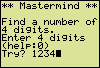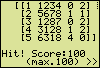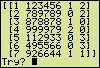# Masterminda program for the Sharp EL-9600, EL-9650and EL-9900 graphing calculators

### A Mastermind game based on numerals instead of colors

Play the familiar game Mastermind with numerals instead of colors and have a hunt for a secret number. The random number is generated by your calculator. Available 'colors' are the numerals from 1 to 9.

To find a four digit secret number the first attempt is for instance 1234.
According to the returned response [1 1234 0 2] no numeral is found to be at the right position while two numerals form part of the hidden number at other locations.The second examination 5678 leads to [1 1], meaning one right numeral at the right position and one right numeral at a wrong position. From both responses it follows that the number in demand holds no nines.
After four attempts the possible numbers left are 6318, 2358 and 2368. Getting the desired outcome [4 0] at the next attempt is only a matter of being in luck...(TI images. The screen examples
differ slightly from Sharp pictures)This Mastermind game can be played at different levels of difficulty. Just make the program generate a random number with more or less digits. To do so, put in a "0", press Enter more than once to pass through some help screens and select 3, 4, 5, 6, 7 or 8 digits.
The option "Resume" enables you to continue a running game. "Stop" (0) will end the running program (MASTRMND).

In case of a six digit number the game could pass as follows.The first two outcomes indicate a double or triple presence of a numeral in the unknown number. After seven examinations only four possible numbers remain:
951471 (double 1), 943375 and 963375 (double 3), and 953275 (double 5).
Evaluating 943375 will result in [2 2], [6 0], [5 0] or [4 0], so it's a hit or the final decision is within close reach.Memory overflow
During the course of a game the amount of stored data increases. To prevent a memory overflow error message from spoiling your game, be sure your calculator has sufficient free memory. In most cases a "Remain" of 2000 bytes will do. A check of the amount of free memory is only a few keystrokes away:
> 2nd,OPTION > MEMCHK > Remain.

Have a nice playing time.

### Program Listing

[ Remarks ]
MASTRMND

Rem MEM IJKZ mat ABC
Rem VER200410
[ > 2ndF,PRGM > PRGM > Rem ]
[ > MATRIX > NAME > mat A ]
ClrT
[ > 2ndF,PRGM > SCRN > ClrT ]
Print "** MASTERMIND **
Print "
Print "BE SURE FSE IS
Print "SET TO FLOATPT
Print "
Print "(SEE SET UP)
Print "
Print ">>
[ > MATH > INEQ > ">" ]
Wait
4→K
[ → : STO-key ]

Label A0
[ > 2ndF,PRGM > BRNCH > Label ]
[ A0, not AO ]
int K→K
[ > MATH > NUM > int ]
min(max(3,K),8)→K
int (1+9rnd_mat(4,K))→mat A
[ > MATRIX > OPE > rnd_mat( ]
K+2→Z
(ln(396/391)/ln 2)^.5→J
[ ^ : ab-key ]
(1–1.5J)/(1–J)*Z→mat A(4,1)
(1.5Z–mat A(4,1))^2/ln 2→mat A(4,2)
{1,4}→dim(mat B)
fill(0,mat B)
Label A1
ClrT
Print "** MASTERMIND **
Print "
Print "FIND A NUMBER OF
Print K
Print "DIGITS.
Print "ENTER DIGITS. (HELP:0)
Label A2
0→Z
Input Z
abs(Z)→Z
If Z<.1*10^K Goto C0
[ mark the decimal point ]
(Z–fPart Z)/10^K→Z
Z–int Z→Z
Z*10^K→mat B(1,2)
0→mat B(1,3)
0→mat B(1,4)
Z→mat A(4,3)
row_mult(0,mat A,1)→mat A
[ > MATRIX > OPE > row_mult( ]
row_plus(mat A,3,1)→mat A
0→J
Label A3
J+1→J
10mat A(4,3)→mat A(4,3)
int mat A(4,3)→mat A(2,J)
If mat A(2,J)=0 Goto C0
mat A(4,3)–mat A(2,J)→mat A(4,3)
If J<K Goto A3
0→J

Label B0
J+1→J
J→I
If mat A(1,J)≠mat A(2,I)Goto B1
mat B(1,3)+1→mat B(1,3)
-1→mat A(1,J)
[ negation symbol (-),
[ not subtraction symbol ]
-2→mat A(2,I)
Label B1
If J<KGoto B0
0→J

Label B2
J+1→J
0→I
If mat A(1,J)=-1Goto B5
Label B3
I+1→I
If mat A(1,J)≠mat A(2,I)Goto B4
mat B(1,4)+1→mat B(1,4)
-1→mat A(1,J)
-2→mat A(2,I)
K→I
Label B4
If I<K Goto B3
Label B5
If J<K Goto B2

mat B(1,1)+1→mat B(1,1)
If mat B(1,1)=1 Goto B6
trans augment(trans mat C,trans mat B)→mat C
[ > MATRIX > MATH > trans ]
Goto B7
Label B6
mat B→mat C
Label B7
ClrT
Print mat C
If mat B(1,3)<K Goto A2
Print "HIT! SCORE (MAX.100):
mat A(4,1)–mat B(1,1)→Z
e^(Z*abs(Z)/mat A(4,2))→Z
[ e^ : 2nd,ex ]
min(Z,1)→Z
(mat B(1,3)+.7mat B(1,4))/K→J
10+90*(.56J+.44Z)*J→Z
int((Z+.5)/5)*5→Z
Print Z
Wait
Goto A0

Label C0
ClrT
Print "VALID DIGITS: 1-9
Print "
Print "INVALID ATTEMPTS
Print "(EXAMPLES):
Print "3303, 0003, 3
Print " Print ">>
Wait :ClrT
Print "RESPONSE:
Print "1 ATTEMPT NUMBER
Print "  NUMBER OF RIGHT
[ 2 spaces in front of "NUMBER" ]
Print "  DIGITS AT
[ 2 spaces in front of "DIGITS" ]
Print "3 RIGHT POSITION
Print "4 WRONG POSITION
Print ">>
Wait :ClrT
Print "CHANGE NUMBER OF
Print "POSITIONS: 3-8
Print "STOP:0       RESUME:
[ 7 spaces in front of "RESUME:" ]
Print K
Print "INFO: WWW.TENHORN.COM
Print "
K→Z:Input Z
ClrT
If Z=0 Goto C1
If (Z=K) and (mat B(1,1)>0) Goto C2
[ > MATH > LOGIC > and ]
Z→K
Goto A0
Label C1
{1,1}→dim(mat C)
mat C→mat A
mat C→mat B
Print "PRESS CL
End
Label C2
Print mat C
Goto A2

If you need help with programming your calculator, this exercise will put you on the way: Creating a Program for the Sharp EL calculator

Hein ten Horn
Top | Programs Sharp EL | Home